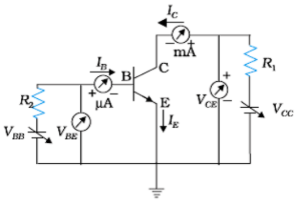(i) Write the three functions of three segments of a transistor.                         (3 marks)

(ii) Draw the circuit diagram for studying the input and output

characteristics of n-p-n transistor in common emitter configuration.

Using the circuit, explain how input, output characteristics are obtained.

(i) Emitter: Supplies the large number of majority charge carries for the

flow of current through the transistor.                                                 (1/2 mark)

Base: Controls the movement of charge carriers coming from emitter

region.                                                                                             (1/2 mark)

Collector: Collects a major portion of the majority carriers supplied

by the emitter.                                                                                  (1/2 mark)

Emitter: Heavily doped and of moderate size.

Base: Central region, thin and lightly doped.

Collector: Moderately doped and large sized.

(ii)(1 mark)

Input characteristics are obtained by recording the values of base current ${\mathrm{I}}_{\mathrm{B}}$,

for different values of ${\mathrm{V}}_{\mathrm{BE}}$ at constant ${\mathrm{V}}_{\mathrm{CE}}$

Output characteristics are obtained by recording the values of ${\mathrm{I}}_{\mathrm{C}}$ for different

values of ${\mathrm{V}}_{\mathrm{CE}}$ at constant ${\mathrm{I}}_{\mathrm{B}}$                                                     (1/2 mark)

[Alternatively

Also accept input/output characteristics curves for this part of the

question.]

Difficulty Level: